8. Solve for x:
7. Solve for x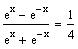a) sketch the graph of f(x)

b) find the domain, range and asysmptotes (if any) of f(x)

c) find the inverse of f(x)
6. Let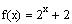5. If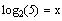and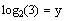, find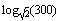Let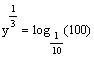. Find the value of y
4.
Write as a single logarithm and simplify your answer: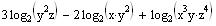3.
Find the solution set of the function2.
a) sketch the graph of f(x)

b) find the domain, range and asysmptotes (if any) of f(x)

c) find the inverse of f(x)
Let1.
Answer all questions. Show all your work
List #.:
Section #.:
Student ID:
Name:
Feb. 25, 2005
Major Quiz 1
Math 002-042